## 深度学习、信息论与统计学 2017-01-06

### 常见的几个概念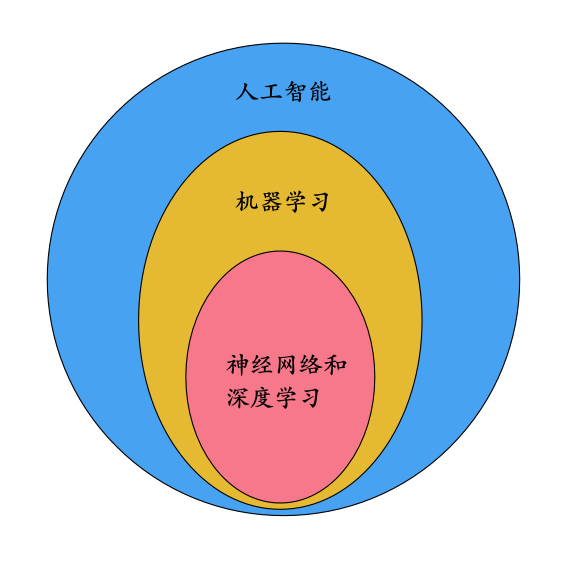### 为什么要用概率来描述？

• 内在的随机性(Inherent stochasticity)。比如，量子力学认为微观世界里的现象是真正的随机现象，而随机性是事物固有的、内在的特性。那么，有没有可能存在一种比量子力学更本质的理论，却是基于确定性的描述呢？这个问题我们恐怕暂时没有能力回答，这属于哲学或者科幻的范畴了。但是，根据主流科学家的观点，我们可以暂且认为真正意义上的随机是存在的，这并不妨碍我们基于概率对一些问题进行建模。
• 不完全观测(Incomplete observability)。比如我让你猜硬币在我的左手还是右手里。对于我来说，我当然很确定地知道它在哪只手里，但在你看来，结果却是不确定的。因为你没有我了解的信息多。
• 不完全建模(Incomplete modeling)。指的是我们的模型（可能为了简单实用）忽略了真实世界的一些信息，所以造成了不确定性。

### 经典统计学的观点

• m个样本组成的数据集记为矩阵X={x(1), x(2), …, x(m)}，对应的mlabel组成的矩阵Y={y(1), y(2), …, y(m)}。
• pdata表示未知的真实的分布(true distribution)，即pdata(y | x)就是我们要估计的总体条件分布。按照经典的统计学，上面m个样本也都是随机变量，它们与pdata(y | x)独立同分布。
• pmodel(y | x;θ)表示一个概率分布族，是我们定义的模型，用于估计真实的概率分布pdata(y | x)。它含有未知参数θ（在神经网络中，参数θ代表了权重w和偏置b），我们机器学习的任务就是估计合适的θ值，让pmodel(y | x;θ)尽可能逼近真实的数据分布pdata(y | x)。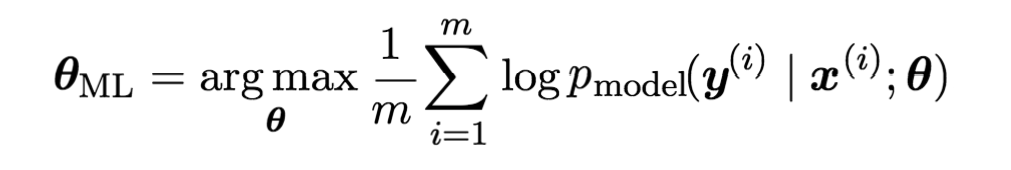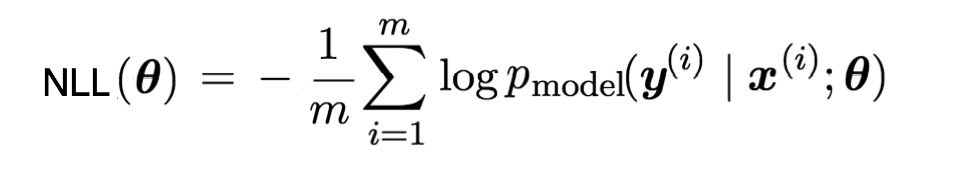### 与信息论的关系

• dog
• cat
• fish
• bird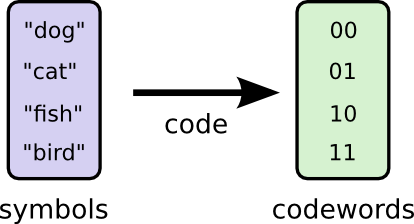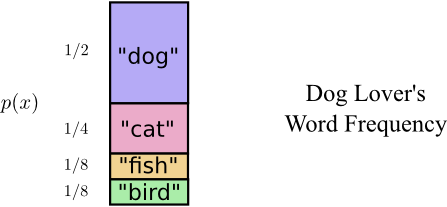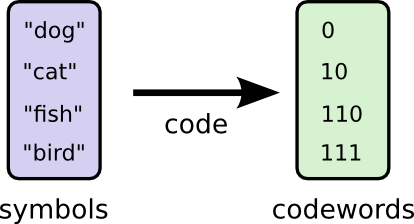I(x) = log (1/p(x)) = -log p(x)

I = -log2 (1/2) = 1bit

H(p) = Ex~p[I(x)] = -Ex~p[log p(x)] = -∑x p(x)*log(p(x))

H(p)可以解释为：概率分布p(x)所包含的平均信息量。那么，如果对p(x)的各个可能的随机事件进行二进制编码，那么平均至少也需要这么多个bit。实际上，针对前面的词汇表中各个单词的概率分布，计算H(p)的值恰好也是1.75bit，因此前面给出的这种变长编码就是最优的一种编码了，再也找不到另外一种编码可以让平均编码长度比信息熵还小。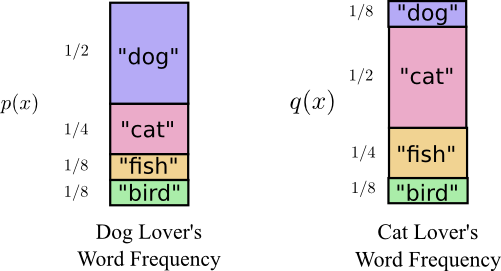H(q,p) = -Ex~q[log p(x)] = -∑x q(x)*log(p(x))

H(q,p)可以理解为：对于符合概率分布q(x)的词汇表使用p(x)对应的最优编码时所得到的平均编码长度。当然，这时对于q(x)来讲就肯定算不上最优编码了。

H(q) = -∑x q(x)*log(q(x)) = -1/8 * log(1/8) - 1/2 * log(1/2) - 1/4 * log(1/4) - 1/8 * log(1/8) = 1.75bit。

Cross-Entropy与Entropy的差，定义了另外一个概念，叫做Kullback-Leibler (KL) divergence。

DKL(q||p) = H(q,p) - H(q) = -Ex~q[log p(x)] + Ex~q[log q(x)]

DKL(q||p) = H(q,p) - H(q) = 2.25bit - 1.75bit = 0.5bit

DKL(pdata||pmodel) = -Ex~pdata[log pmodel(y|x;θ)] + Ex~pdata[log pdata]

H(pdata, pmodel) = -Ex~pdata[log pmodel(y|x;θ)]H(pdata, pmodel) = NLL(θ)

• 最大似然估计
• 使NLL最小化
• 使KL divergence最小化
• 使Cross-Entropy最小化

### 贝叶斯统计的观点

• 在贝叶斯统计中，任何未知的变量都能看成是随机变量。
• 贝叶斯统计有一个比较“主观”的先验分布的概念。

p(θ | x) = p(x | θ) * p(θ) / p(x)

log p(θ | x) = log p(x | θ) + log p(θ) - log p(x)

### 一些常见的Cost Function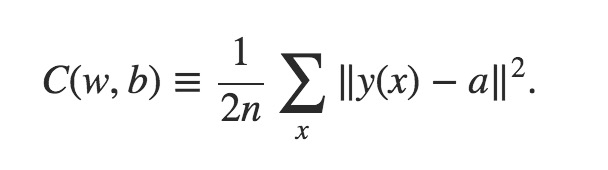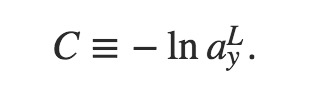• 如果将pmodel看成一个高斯分布（即正态分布），而且输出单元是线性输出，就能推导出MSE的形式。
• 如果将输出层的每一个输出单元都看成是Bernoulli分布（即0-1分布），就能推导出所谓的”Cross-Entropy Cost Function”。这时输出单元一般是Sigmoid神经元。
• 如果将输出层的多个输出看成是Multinoulli分布（也称为Categorical分布），即典型的分类器场景，那么就能推导出log-likelihood形式的Cost Function。这时输出单元一般采用softmax形式。### 小结

• 这个基础告诉我们应该尽力优化pdatapmodel之间的差异，但pdata和真正的pdata之间的差异却不是这个基础能决定的。这取决于样本数据的质量。
• pmodel本身的设计只能凭人的经验，而没有坚实的理论支持来指导我们如何确定它。
• 贝叶斯推断引入的对于未知参数的先验分布，仍然主要依赖人的经验。

（完）

##### 参考文献：